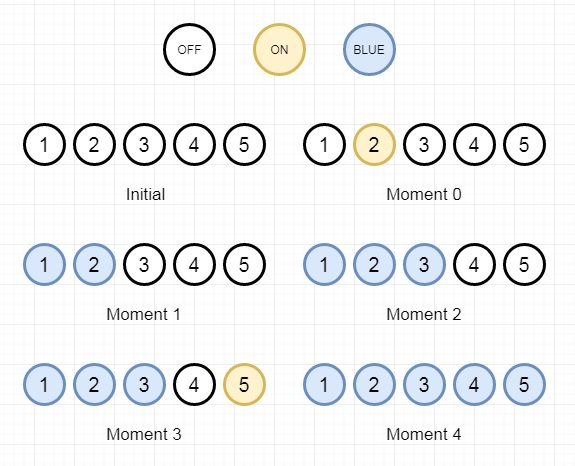# Bulb Switcher III in C++

Suppose we have a room with n bulbs, these are numbered from 1 to n, arranged in a row from left to right. Initially, all the bulbs are turned off. At moment k (for k in range 0 to n - 1), we turn on the light[k] bulb. A bulb changes color to blue only if it is on and all the previous bulbs (to the left) are turned on too. We have to find the number of moments in which all turned on bulbs is blue. So this is an example −The output will be 3 as the moments are 1, 2 and 4.

To solve this, we will follow these steps −

• ret := 0, define a set x, n := size of list array, define a map m

• define a max heap-based priority queue pq

• for I in range 0 to n – 1

• m[light[i]] := i and insert i into pq

• for I in range 1 to n

• insert m[i] into x

• while pq is not empty and top element of pq is in the set x,

• delete from pq

• ret := ret + 1 when (pq is empty or top of pq >= i), otherwise 0

• return res

## Example (C++)

Let us see the following implementation to get a better understanding −

Live Demo

#include <bits/stdc++.h>
using namespace std;
class Solution {
public:
int numTimesAllBlue(vector<int>& light) {
int ret = 0;
set <int> x;
int n = light.size();
map <int, int> m;
priority_queue <int, vector <int>, greater <int> > pq;
for(int i = 0; i < n; i++){
m[light[i]] = i;
pq.push(i);
}
for(int i = 1; i <=n; i++){
x.insert(m[i]);
while(!pq.empty() && x.count(pq.top()))pq.pop();
ret += (pq.empty() || (pq.top() >= i));
}
return ret;
}
};
main(){
vector<int> v = {2,1,3,5,4};
Solution ob;
cout << (ob.numTimesAllBlue(v));
}

## Input

[2,1,3,5,4]

## Output

3

Updated on: 29-Apr-2020

240 Views